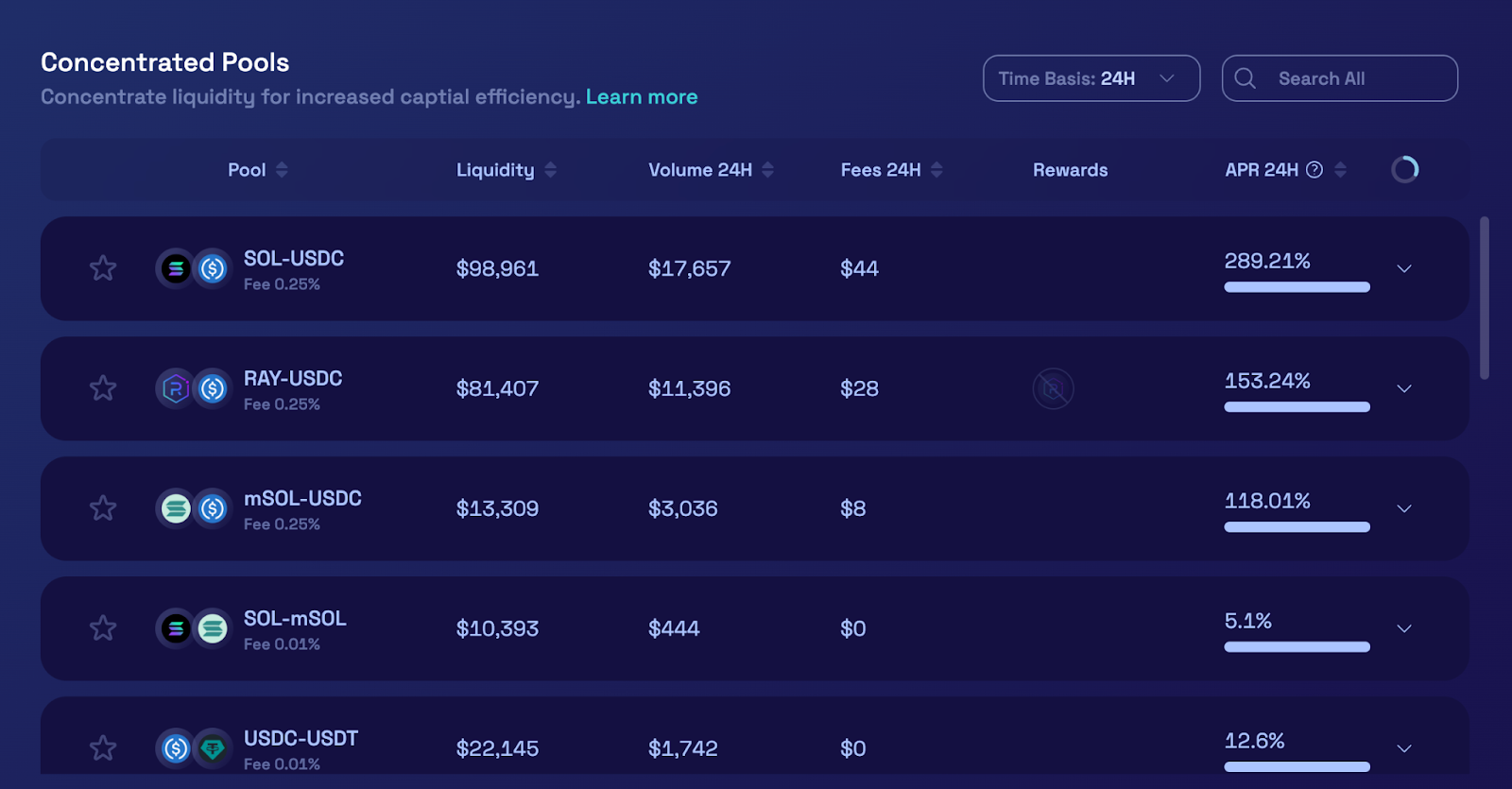Comment on page

# Estimated APR Calculations

### APR Calculations for CLMM pools

In a CLMM pool, when price trades within an individual tick, fees are distributed proportionally to the liquidity provided by in-range positions. An accurate APR can be found for an individual tick based on trading volume within a time period, however, extrapolating this to all of the ticks in the pool and among multiple LPs is extremely complex. Traditional constant product pool APR calculations can not be directly applied to CLMM pools. Projected returns for CLMMs should be considered as an estimate at best.
There are three methods for estimating APRs for CLMM pools displayed on Raydium, each with its own calculation described in the following sections:
1. 1.
Overall Pool Estimated APR
2. 2.
Estimated APR for a user position (two methods of calculation below)
• Delta Method
• Multiplier Method

### Overall Pool Estimated APR

In order to estimate an overall APR for the pool, the following is assumed: Trading fees and emissions per block are extrapolated across all of the liquidity in the pool, including liquidity positions that are out of range.### Delta Method - Estimated APR for user positions

The Delta Method applies the implied change (delta) in pool liquidity, as determined by a user specified position price range and size, to calculate an estimated APR.
The total amounts of each token can be calculated by using the following formulas:For estimation of the amounts of tokenA (deltaX) and tokenB (deltaY) we need to know deltaL:After calculating for deltaL, we can calculate deltaX and deltaY using:Estimated daily fee can be calculated by:And can be calculated from:### Multiplier Method - Estimated APR for user positions

The Multiplier Method applies a multiplier, determined by the intersection of the position price range and the historical price range of the pool to calculate an estimated APR.
In order to estimate the future reward and fee APR, the following is assumed:
• Historical price data is used to extrapolate future price data, practically this is not the best indicator of future price performance but it does help to provide a decent estimate.
• Price fluctuation within the historical price range is consistent in volume across the entire time interval, and resembles a periodic function with an amplitude equalling the magnitude of the upper and lower price boundaries.
We retrieve the following variables:For ease of understanding, we will denote the following ranges as such:Where retroRange is the retroactive intersection between userRange and histRange